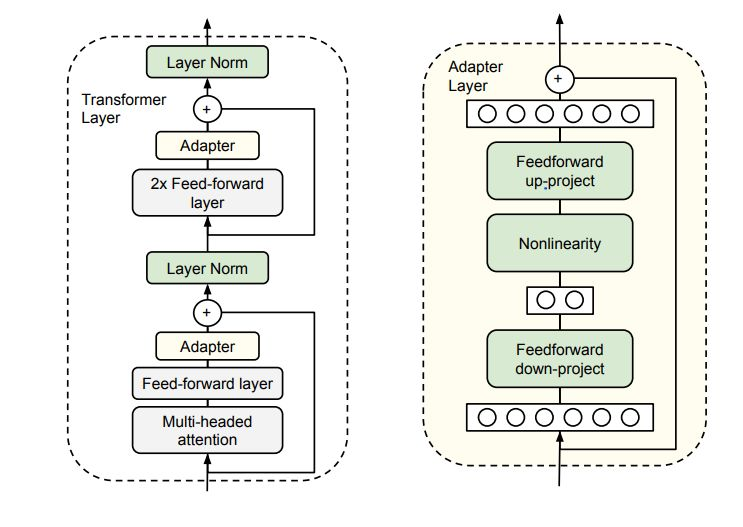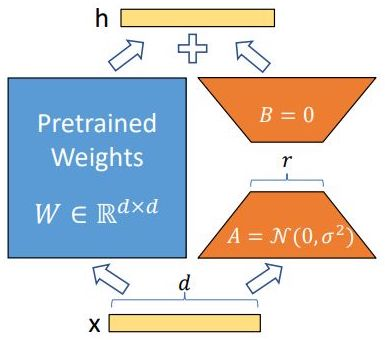## Overview

• Fine-tuning of large pre-trained models on downstream tasks is called “transfer learning”.
• While full fine-tuning pre-trained models on downstream tasks is a common, effective approach, it is an inefficient approach to transfer learning.
• The simplest way out for efficient fine-tuning could be to freeze the networks’ lower layers and adapt only the top ones to specific tasks.
• In this article, we’ll explore PEFT (Parameter Efficient Fine-Tuning) methods that enable us to adapt a pre-trained model to downstream tasks more efficiently – in a way that trains lesser parameters and hence saves cost and training time, while also yielding performance similar to full fine-tuning.

• Parameter-efficient fine-tuning is useful due the following reasons:
1. Reduced computational costs (requires fewer GPUs and GPU time)
2. Faster training times (finishes training faster)
3. Lower hardware requirements (works with cheaper GPUs with less VRAM)
4. Better modeling performance (reduces overfitting)
5. Less storage (majority of weights can be shared across different tasks

• Adapters is a PEFT (Parameter Efficient Fine-tuning) technique shown to achieve similar performance as compared to tuning the top layers while requiring as fewer parameters as two orders of magnitude.
• Adapter-based tuning simply inserts new modules called “adapter modules” between the layers of the pre-trained network. Keeping the full PT model frozen, these modules are the only optimizable ones while fine-tuning - this means only a very few parameters are introduced per task yielding “compact” models.

### What is an Adapter Module?

• Let’s look at the application of the adapter module in the transformer architecture in three points:
• The adapter module (right) first projects the original $$d$$-dimensional features into a smaller $$m$$-dimensional vector, applies a nonlinearity, and then projects it back to $$d$$ dimensions.
• As can be seen, the module features a skip-connection - With it in place, when the parameters of the projection layers are initialized to near-zero which eventually leads to near identity initialization of the module. This is required for stable fine-tuning and is intuitive as with it, we essentially do not disturb the learning from pre-training.
• In a transformer block (left), the adapter is applied directly to the outputs of each of the layers (attention and feedforward).

### How to decide the value of $$m$$?

• The size $$m$$ in the Adapter module determines the no. of optimizable parameters and hence poses a parameter vs performance tradeoff.
• The original paper experimentally investigates that the performance remains fairly stable across varying Adapter sizes m and hence for a given model a fixed size can be used for all downstream tasks.• Looking to avoid high GPU costs when fine-tuning a model?
• The basic idea behind LoRA is:

Heavily Parameterized Large Language Models + Basic Linear Algebra Theorem = Save GPU memory!

• The downsides of some of the other fine-tuning techniques for multitask learning are:
• Adapters: introduces inference latency that becomes significant in online low batch size inference settings.
• Prefix tuning: reduces the model’s usable sequence length.
• LoRA (low rank adaptation) is a PEFT (parameter efficient fine-tuning) technique that relies on a simple concept - decomposition of non-full rank matrices.
• LoRA hypothesizes that “change in weights” during adaptation has a “low intrinsic rank”. $$\Delta W$$ is non-full rank and so can be written as $$\Delta W = BA$$ (c.f. figure below).
• A matrix is said to be rank-deficient if it does not have full rank. The rank deficiency of a matrix is the difference between the lesser of the number of rows and columns, and the rank. For more, refer Wikipedia: Rank.• “Low intrinsic rank” is inspired by the idea of “low intrinsic dimensionality” that these over-parameterized pre-trained models are seen to reside on, and that’s also the explanation behind why fine-tuning only a part of the full model rather than full fine-tuning can yield good results.
• During training, the outputs from $$W$$ and $$\Delta W$$ are added component wise, like so:
$h = Wx + BAx$
• All we’re now left to optimize is the new matrices $$B$$ and $$A$$ that contain a very smaller number of parameters (combined) than the full matrix due to their dimensions.
• In summary, all of the pre-trained weights W are kept frozen and the rank decomposition matrices of the “change in weight matrix”, $$B$$ and $$A$$, are optimized.
• This yields significant benefits as compared to full-fine tuning:
• Time and memory efficiency: With a large percentage of the parameters being frozen, the training time and the GPU memory is saved. Saving is more when using stateful optimizers like Adam, Adadelta, etc.
• Storage efficiency: No need to store huge checkpoints for different downstream tasks. Checkpoint size is greatly reduced with reduction in trainable parameters.
• Easy task-switching in deployment: all we need to change is a handful of weights as compared to the full model.
• Results:
• With GPT-3 175B, the VRAM consumption during training is reduced from 1.2TB to 350GB, and the trained checkpoint size reduced from 350GB to 35MB!!!
• LoRA achieves performances comparable to and sometimes even better than fine-tuning the full model.

## Citation

If you found our work useful, please cite it as:

@article{Chadha2020DistilledMultitaskLearning,